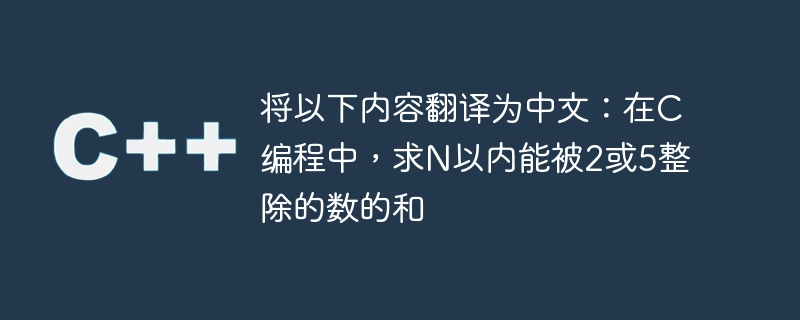# 将以下内容翻译为中文：在C编程中，求N以内能被2或5整除的数的和WBOY 转载
2023-09-20 08:25:06 842浏览`Sum2 = ((n / 2) * (4 + (n / 2 - 1) * 2)) / 2`

`Sum5 = ((n / 5) * (10 + (n / 5 - 1) * 5)) / 2`

`Sum10 = ((n / 10) * (20 + (n / 10 - 1) * 10)) / 2`

`Sum = Sum2 + Sum5 - Sum10`

## 示例

```#include <stdio.h>
int main() {
int n = 25;
long int sum2, sum5, sum10;
sum2 = ((n / 2) * (4 + (n / 2 - 1) * 2)) / 2;
sum5 = ((n / 5) * (10 + (n / 5 - 1) * 5)) / 2;
sum10 = ((n / 10) * (20 + (n / 10 - 1) * 10)) / 2;
long int sum = sum2 + sum5 - sum10;
printf("Sum is %d", sum);
return 0;
}```

## 输出

`Sum is 201`